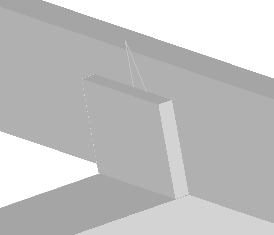Command -  Prb_MoveRotate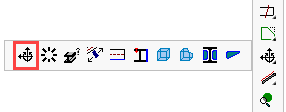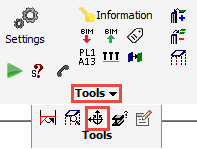This tool allows one to directly change the distance between 2 parts, or to set a rotation between 2 parts.

The uniqueness of this tool is that it works on sub geometries for the input.

Counterpart commands such as move and rotate will work on coordinates instead of geometries.

In order for this dialog box to work, we need to select at least 1 geometry under the fixed geometry, and select 1 geometry under the flexible geometry.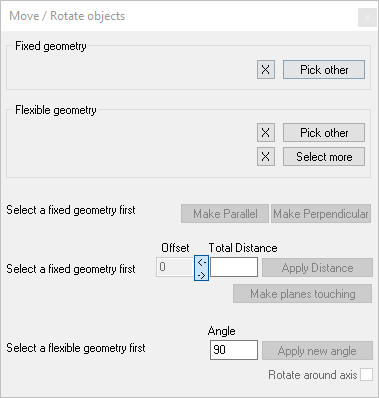A short description for each option in this dialog :

Fixed Geometry

This is the reference geometry that will not be moved or rotated.

This would be the geometry to which you may want to align the flexible geometry to.

This geometry can be a point, a line or any planar surface of a Parabuild part.

Flexible Geometry

This is the geometry that will be moved and/or rotated to satisfy the distance or angle action (see below).

This geometry can be a point, a line or any planar surface of a Parabuild part.

You can use the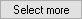button to add more objects to the selection set. All of these extra objects will join in the same move/rotate operations.

Make parallel and Make Perpendicular

These buttons will only do a rotate operation to make both flexible geometry parallel or perpendicular with the fixed geometry

Offset

Use this only if you want to move the part(s) in the direction of the Fixed geometry, while ignoring the current distance between the parts.

Total distance

This value will be filled with the current distance between the parts.
This value can thus be seen as a measurement.

However this value may be misleading when the fixed and flexible geometries are not parallel.

Apply Distance

This action will move and rotate the Flexible geometry to satisfy either the Offset value or the Total Distance value (whichever value is active).

• In case of the Offset value a simple move operation will be done in the direction of the Fixed geometry
• In case of the Total Distance value the flexible geometry is moved and rotated so that the gap between the parts is the distance entered here.

Make planes touching

This does almost the same as Apply Distance, but the distance will be 0, and the flexible part is rotated so that the material directions are opposed so that the parts are not colliding.

Apply new angle

This will do a rotation only.

It will rotate the Flexible geometry around the fixed geometry.

If you selected planes for both the fixed and the flexible geometry, then the flexible geometry will rotate with the plane's normal as the axis of the rotation.

The value in the angle field is not filled in automatically so this shouldn't be seen as a measurement.

Example

This is an example move operation that can be achieved with the Apply distance button.

This is the issue that needs to be solved : the plate was drawn relative to World but instead it should follow the roof's pitch :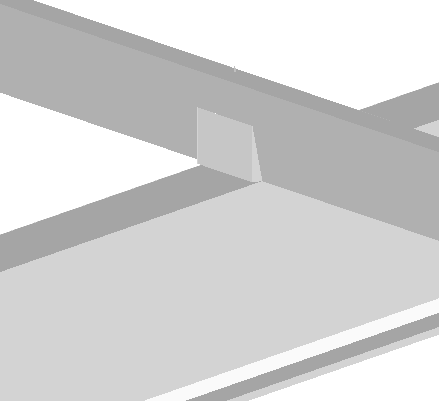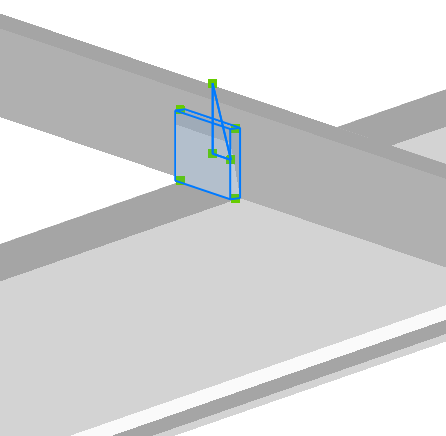Selecting the fixed plane :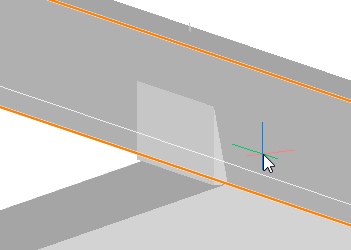Selecting the plane that needs to be rotated :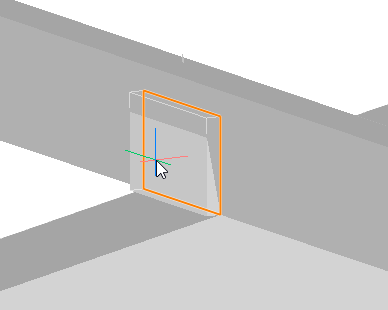The end result after applying a Total Distance of 0 :